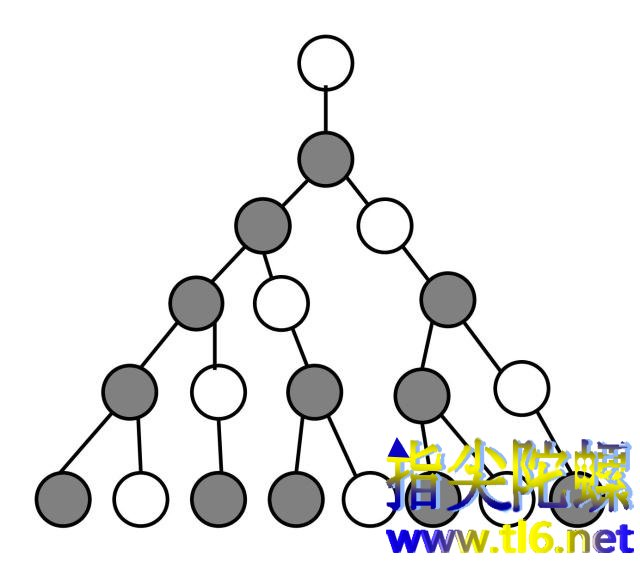1,1, 2, 3, 5, 8, 13, 21, 34, 55, ···

0, 1, 1, 2, 3, 5, 8, 13, 21, 34, 55, ···

Ln =Fn-1 +Fn+1,  其中 n=1,2,3, ···

L1 =F0 +F2 = 0+ 1=1

L2 =F1 +F3 = 1+ 2=3

L3 =F2 +F4 = 1+ 3=4

L4 =F3 +F5 = 2+ 5=7

L5 =F4 +F6 = 3+ 8=11

L6 =F5 +F7 = 5+ 13=18

L7 =F6 +F8 = 8+ 21=29

L8 =F7 +F9 =13+ 34=47

L9 = F8 +F10 =21+ 55=76

L10 =F9 + F11 =34+ 89=123

L11 =F10 +F12 =55+144=199

L12 =F11 +F13 =89+233=322

L13 =F12 +F14 =144+377=521

............

1,3,4,7,11,18,29,47,76,123,199,332,521,···

Ln =Fn-1 +Fn+1

=(Fn-3 +Fn-2 ) +(Fn-1 +Fn )

=(Fn-3 +Fn-1 ) +( Fn-2 + Fn )

=Ln-2+Ln-1

L2－1=3－1=2=1×2

L3－1= 4－1 = 3 = 1×3

L5－1= 11－1 =10=2×5

L7－1= 29－1 =28=4×7

L11－1= 199－1 =198=18×11

L13－1 = 521－1 =520 =40×13